cancel
Showing results for
Did you mean:

Fabric is Generally Available. Browse Fabric Presentations. Work towards your Fabric certification with the Cloud Skills Challenge.## Unveiling Top Products with categories: A Guide to GENERATE and TOPN Functions

Let's consider the following task: within one table, let's place categories by products and for each category let's place the best-selling product in this table.

To solve the problem, we use the GENERATE function. This function creates a table from all intersections of rows of the two tables included in the function parameters. That is, for each row of the first table, all rows from the second table are returned in turn. In our case, the first table will be the product category table and the second table will be the table with products. However, we will need to return not all products for each category, but only the top one. In turn, to get the top product, the table must be filtered using the TOPN function.

First, let's familiarize ourselves with the GENERATE function using a simple example, and then we'll move on to our more complicated example.

There are two tables that are not related to each other. One table with product namesSecond table with managersLet's merge the tables using the GENERATE function. Go to modeling and create a table - to do this, write GENERATE. Where we specify the first table as the first parameter, and the second parameter - the second table.

We are returned a combined table by rows and by columns. For each row from the first table, all rows from the second table are added, etc.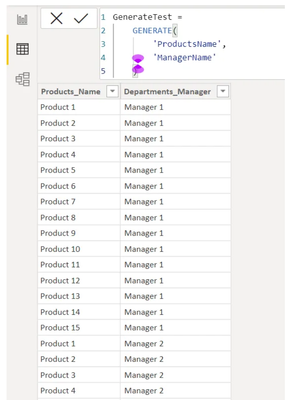We can also join tables with multiple columns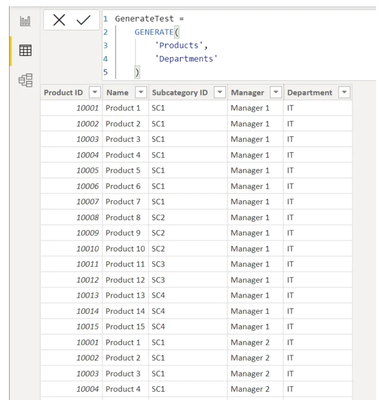Let's take our data model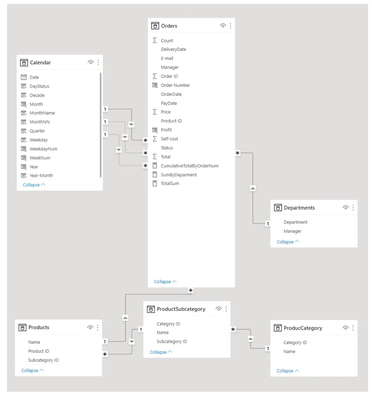The goal of the task is as follows: we need to output the name of the top product for each category and we need to do it within one table. Let's consider our tables: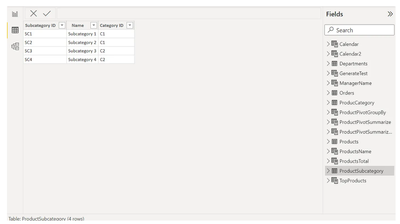Let's start creating the corresponding table. Go to the modeling tab. Create the table: write GENERATE, specify the Products directory in the first parameter, specify the ProductCategory directory in the second parameter.

Let's see what we got: a rather large table. For each row from the first table we have returned all rows and glued to the second table, as well as for all other rows of the table. As a result, we have 30 rows.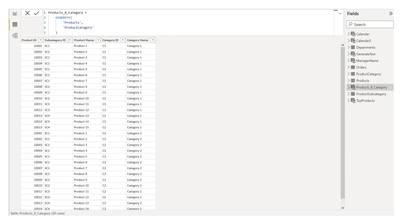This is redundant for us. Therefore, in order to keep only those training courses that correspond to the categories, we need to filter the products by the category string. We could use the FILTER function, but it only allows us to specify a static filter. Therefore, according to the relationship between the tables, we use RELATEDTABLE.Now we need to display not all products for each category, but only the top selling ones. The TOPN function is responsible for selecting the top and it turns out that we need to filter this table by product. Let's wrap the RELATEDTABLE function with the help of TOPN, and in the first parameter we specify the number 1, in the second parameter there is a table on which we need to output the top-1, in the third parameter we need to specify the column on which the top will be displayed - it is not present at the moment, so we will add it through the ADDCOLUMNS function.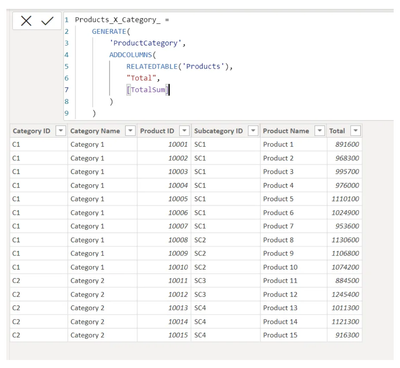In the first parameter of the ADDCOLUMNS function we supply a table with products, in the second and third parameters we create a corresponding column. We name this column, e.g. Total, and use the measure to calculate this column.Alternatively, we can count the Total column inside the calculated table. To do this, we need to filter the table with two filters: filter by specific product and filter by account status.

Let's calculate the Total column from the Orders table using the SUMX function, because we can insert a table into the SUMX function and this table can be filtered using the FILTER function. We filter the Orders table by Status = ACCEPTED. In the second parameter of the SUMX function we insert the Total column and the function will calculate the sum of this column.

It turns out that the SUMX function will calculate the Total column for each productDon't forget that the TOPN function is not finished yet, we specify in the third parameter the column by which we will summarize the top, in our case it is the Total column, it was created using ADDCOLUMNS.

The last parameter in the TOPN function is the order from where to count the top, choose DESC.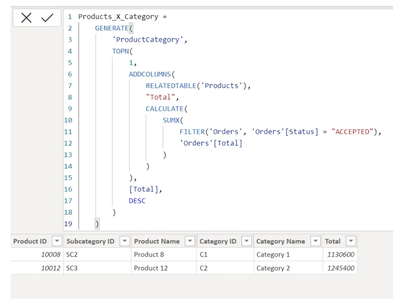If we want, we can show the top three products per categoryThe value of each product was passed to the Orders table using RELATEDTABLE and as a result Orders was filtered by product and by order status - ACCEPTED. After that, the SUMX function calculated the Total amount and the TOPN function calculated the top 3 products for each category.

This table contains too many unnecessary columns, we need three columns: Category Name, Product Name and Total. These three columns can be selected using the SELECTCOLLUMNS function.

Let's wrap the whole GENERATE table and as the first parameter we give the table that the GENERATE function returns, as the next parameters we write 3 columns, each of which can be named.

The first column we call Category and refer to the Category Name column, the second column we call Product and refer to the corresponding Product Name column, the third column Total, we refer to a virtual column, that is, not through the table, but through a square bracket, because this column was created in the code of the table.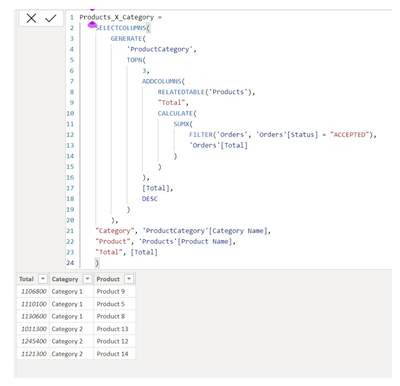Now for each category the top 3 products are considered and you can build a report on the basis of this table.

To get a table with columns in the required order, it is necessary to delete and recreate this table.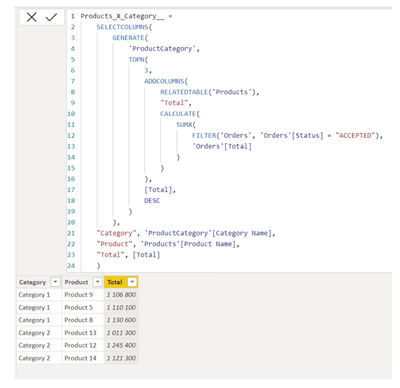Can someone help with the power bi DAX below.  It is giving me the following error

Expression.Error: The name 'IF' wasn't recognized.

IF(Category = "Domestic",
IF(A1 <= 5, A1 * 2100, IF(A1 <= 40, 5 * 2100 + (A1 - 5) * 2250, 5 * 2100 + 35 * 2250 + (A1 - 40) * 2540)),
IF(Category = "Institutional",
IF(A1 <= 5, A1 * 2030, IF(A1 <= 40, 5 * 2030 + (A1 - 5) * 2360, 5 * 2030 + 35 * 2360 + (A1 - 40) * 2540)),
IF(Category = "Commercial", A1 * 1930,
IF(Category = "Industrial", A1 * 2190,
IF(Category = "Kioks", A1 * 1240,
IF(Category = "WAT", A1 * 2300, 0)
)
)
)
)
)@jacksonomondi this task would be better placed in the DAX section:

Top Kudoed Posts
Latest Articles